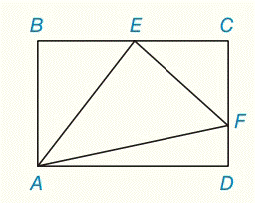Chapter 5.CR, Problem 33CR### Elementary Geometry for College St...

6th Edition
Daniel C. Alexander + 1 other
ISBN: 9781285195698

#### Solutions

Chapter
Section### Elementary Geometry for College St...

6th Edition
Daniel C. Alexander + 1 other
ISBN: 9781285195698
Textbook Problem
1 views

# Given: A B C D ​ ​ ​       is     a     rectangle E is the midpoint of  B C ¯ A B = 16 , C F = 9 , A D = 24 Find: A E , E F , A F , m ∠ A E FTo determine

To find:

AE, EF, AF, mAEF.

Explanation

Given:

Calculation:

From ΔBAE

Let us first take the triangle ΔABC

By Pythagoras theorem,

AE2=BA2+BE2AE2=162+122AE2=256+144AE2=400AE=400AE=20

To find EF, consider the triangle ΔECF

By Pythagoras theorem,

EF2=EC2+CF2EF2=122+92EF2=144+81EF2=225EF=225EF=15

By Pythagoras theorem,

### Still sussing out bartleby?

Check out a sample textbook solution.

See a sample solution

#### The Solution to Your Study Problems

Bartleby provides explanations to thousands of textbook problems written by our experts, many with advanced degrees!

Get Started

#### Find more solutions based on key concepts